# Host/Guest Complexes Containing Cyclodextrins and Dyes

By: Daniel Jenkinson

Abstract

It was determined that the wavelength of fluorescence of PRODAN is lengthened in a more polar solvent, or when complexed in a cyclodextrin, since the energy difference between the ground and excited state is lowered due to stabilisation from polar groups. When using β-cyclodextrin, a 1:1 stoichiometry is adopted, but when using γ-cyclodextrin, a 2:1 ratio of PRODAN: cyclodextrin is preferred. The equilibrium constant for bonding with β-cyclodextrin was 8500 M-1, and the product of the two required equilibrium constants when using γ-cyclodextrin was 12,900 M-2.

Introduction

All molecules and atoms have a ground electronic state, along with at least one excited state. The ground state is a singlet state, since all electrons are spin paired. Most atoms and molecules have excited singlet states of higher energy than that of the ground state. Some species have excited triplet states, where electrons are unpaired and have parallel spins. Each excited state has associated vibrational levels.

When a photon of light with energy equal to that of the energy difference between the ground and excited electronic state of a molecule, an electron can absorb the energy and can be promoted to any vibrational level associated with the excited state. Electrons that are promoted to high energy levels lose their energy quickly through collisions with other molecules (for example, with solvent molecules, which is a process known as external conversion). If this does not happen, there are two main pathways for the excited electron to return down to the ground state. The electron can emit a photon of light equivalent in energy to the energy difference between the excited and ground state, which is a process known as chemiluminescence. If the photon is released as a result of an electron relaxing from a singlet state, the light emitted is known as fluorescence. Since this process is spin-allowed, it characteristically happens more quickly (nanosecond scale) than phosphorescence, which is when a photon is released as the result of an electron relaxing to the ground state from a triplet state.[i] These processed can be described using a Jablonski diagram, as shown in figure 1.

Supramolecular chemistry has been defined by the Encarta English Dictionary as chemistry that is more complex in form than a molecule. It encapsulates chemistry where assemblies of molecules are held together with intermolecular forces rather than covalent or ionic bonds. In recent years, Host-Guest chemistry has received a lot of interest and attention, and involves the design and synthesis of “host molecules” with the intent of selectively binding to a specific “guest molecule.”

One type of host molecule that has been widely studied is the cyclodextrin, which are composed of rings of between 5 and 10 glucose molecules. The most commonly encountered cyclodextrins have 6, 7 or 8 glucose molecules in the ring, and are called α-, β- and γ-cyclodextrin. They each adopt a torus shape (like a cone but the spiky bit has been chopped off) where the hydrophilic hydroxyl groups of the glucose molecules point outwards, leaving a hydrophobic interior. This enables the cyclodextrins to encapsulate an enormous number of molecules (driven by the hydrophobic effect, Van Der Waals interactions, and the expulsion of polar solvents from the cavity) and has led to their commercial success in many applications.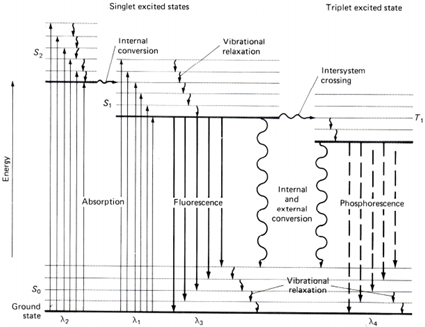Figure 1 – A Jablonski diagram where S0 is the ground state, S1 and S2 are excited singlet states, T1 is an excited triplet state

α-Cyclodextrin was first synthesised in 1984 by simply using an intramolecular reaction of the two ends of a hexamer of glucose. To avoid early cyclysation, one end of the chain is protected.[i] Cyclodextrins can by purified using HPLC.[ii]

Figure 2 – the structural formula of PRODANPRODAN, as shown in figure 2, is a molecule that can both absorb photons of light, and emit them in the process of electron relaxation. It can also fit into the cavity of cyclodextrins. PRODAN is known as a solvochromatic molecule because it has electron donating and electron withdrawing groups in resonant positions around a conjugated system. When PRODAN absorbs a photon, the dipole moment increases as a result of charge travelling along the conjugated system in the process of intramolecular charge transfer, as shown in figure 3.[i] This experiment explores how different solvents alter the wavelength of emitted fluorescence.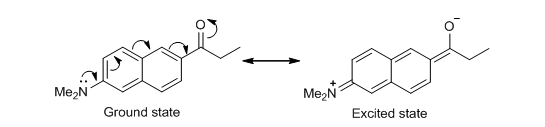Figure 3 – a diagram to show resonance structures of the ground and one example of an electronically excited state of PRODAN via intramolecular charge transferEquation 1 demonstrates how a varying degree of complexation will alter the fluorescence emission by PRODAN, given that the stoichiometry of complexation is 1:1, where F is the intensity of fluorescence at a concentration of cyclodextrin equal to [CD], F0 is the intensity of fluorescence in the absence of cyclodextrin, F is the intensity of fluorescence when all PRODAN is complexed, and K1 is the equilibrium constant of complexation.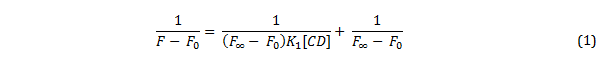Plotting a graph of 1/ (F-F0) against 1/ [CD] gives a straight line which with the equilibrium constant can be derived by dividing the y-intercept by the gradient of the line. However, if a cyclodextrin is capable of complexing more than one molecule of PRODAN, the change in fluorescence intensity is described by equation 2, where K1 is the equilibrium constant for the first complexation, and K2 is the equilibrium constant for the second complexation.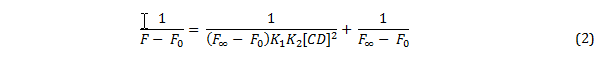If this is the case, then a plot of 1/ (F-F0) against 1/ [CD]2 should yield a straight line, from which the product of the two equilibrium constants can be calculated by dividing the y-intercept by the gradient of the line.

The aims of this experiment were to determine the effect that solvent had on the emitted wavelength of light due to fluorescence; to establish whether a host-guest complexation between PRODAN and β-cyclodextrin has an effect on the relaxation of electrons; to establish the equilibrium constant of the complexation between PRODAN and β-cyclodextrin; and to determine whether cyclodextrin forms complexes to one or two molecules of PRODAN.

Experimental

Solvent dependence of PRODAN fluorescence

The fluorescence emission spectrum between 400 and 600 nm of provided solutions of PRODAN in water, ethanol, DMSO, chloroform and cyclohexene using an excitation frequency of 360 nm in a quartz cuvette. Additional parameters are given in table 1. Each spectrum was recorded to determine the wavelength at which the maximum fluorescence occurs.

Table 1

 Solvent Excitation slit width / nm Emission slit width / nm Photomultiplier voltage Approximate emission intensity Water 10 10 Medium 630 Ethanol 10 10 Low 480 DMSO 20 10 Low 570 CHCl3 20 10 Low 760 Cyclohexane 10 10 Medium 720

Host-guest complexation of PRODAN by β-cyclodextrin

A sample of β-cyclodextrin (1.70 g, 1.5 mmol) was dissolved in Milli Q water (50 ml) with the aid of gentle heating. The solution was then transferred to a 100 ml volumetric flask and made up to the mark with Milli Q water. The solutions in table 2 were made up in 10 ml volumetric flasks using the stock solution of β-cyclodextrin previously prepared, and the supplied solution of 2 x 10-6 M PRODAN in water. The volumetric flasks were filled to the mark using Milli Q water.

Table 2

 Solution Final concentration of PRODAN / µM Volume of stock PRODAN solution required / ml Final concentration of β-cyclodextrin / M Volume of stock β-cyclodextrin solution required / µl A 0.4 2 0.0000 0 B 0.4 2 0.0003 200 C 0.4 2 0.0006 400 D 0.4 2 0.0012 800 E 0.4 2 0.0030 2000 F 0.4 2 0.0060 4000 G 0.4 2 0.0120 8000

The solutions were thoroughly mixed before measuring their emission spectra using the conditions stated below in disposable polymethacrylate cuvettes. The spectrum of solution G was measured first since it had the highest concentration of β-cyclodextrin. The PMT voltage was adjusted until the emission intensity was between 800 and 1000 at 500 nm. After this, the other spectra were recorded to determine their emission intensity at 500 nm.

Fluorescence parameters

Excitation wavelength                 385 nm

EM start wavelength                     400 nm

EM end wavelength                       650 nm

Ex slit                                                   10 nm

EM slit                                                  10 nm

PMT voltage                                      657 V

Results

Solvent dependence of PRODAN fluorescence

Table 3

 Solvent Wavelength of emission / nm Water 524 Ethanol 490 DMSO 465 CHCl3 437 Cyclohexane 394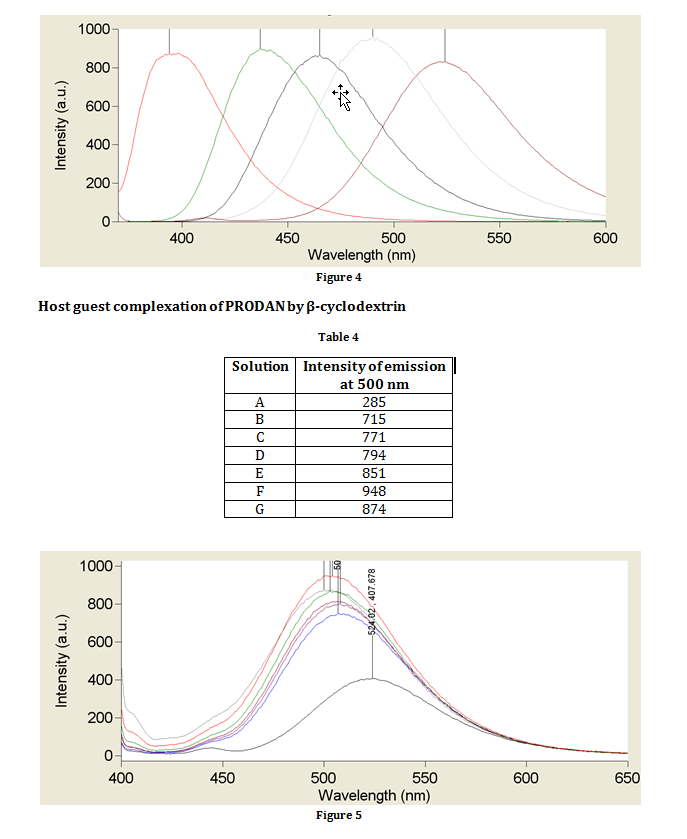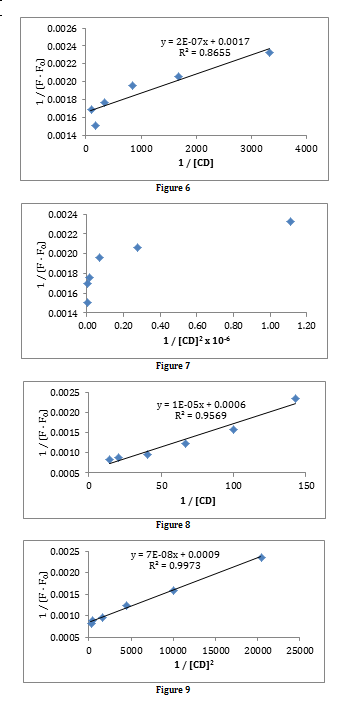Discussion

Solvent dependence of PRODAN fluorescence

As shown in figure 3, the excited state of PRODAN is a zwitterion (a molecule with two oppositely charged centres). Because it is a species with localised charges, the energy of the species can be lowered by using a polar solvent. A more polar solvent lowers the energy of the excited state by stabilising the charges; however, the energy of the ground state remains approximately the same in all solvents. This is reflected in the results obtained. Since energy of a photon is inversely proportional to its wavelength, the most polar solvent (water) which has the greatest stabilising effect causes the PRODAN to emit fluorescence at the longest wavelength, and the least polar solvent (cyclohexene) causes PRODAN to emit fluorescence at the shortest wavelength.

When cyclodextrins are introduced, the wavelength of emitted photons is similar to that of when water is used as a solvent for PRODAN. This is likely to be due to stabilisation of the charges from the many hydroxy groups on the cyclodextrin ring. There is a more dramatic shift in the wavelength of maximum fluorescence when a larger cyclodextrin ring was used because there are more hydroxy groups to stabilise the charges.

Host guest complexation of PRODAN by β-cyclodextrin

Figures 6 and 7 are plots to determine whether the complexation has a 1:1 or a 2:1 stoichiometry, and then to determine the equilibrium constant of complexation. It can be seen that the complexation has a 1:1 stoichiometry since a straight line is obtained in figure 6, but not in figure 7. From the line of best fit in figure 6, the equilibrium constant can be calculated, as stated in the introduction, by dividing the y-intercept by the gradient.

K1 = 0.0017 / 2×10-7

K1 = 8500 dm3 mol-1

This is a very high value, which indicates that the binding affinity between PRODAN and β-cycloxtrin is strong, and that the complexation reaction is thermodynamically favourable.

Data was provided for the complexation between PRODAN and γ-cyclodextrin. Plots to determine the stoichiometry of complexation with γ-cyclodextrin are shown in figures 8 and 9. Both plots produce a straight line, indicating that there is a mixture of both methods of complexation taking place; however, the R2 value for the best fit line in figure 9 is higher than the corresponding value in figure 8. This suggests that a higher degree of complexation is taking place in a 2:1 ratio of PRODAN to cyclodextrin than in a 1:1 ratio, which can be proven by calculating the equilibrium constants for each type of complexation.

K1 = 0.0006 / 1×10-5

K1 = 60 dm3 mol-1

K1K2 = 0.0009 / 7×10-8

K1K2 = 12,900 dm6 mol-2

The product of equilibrium constants calculated from figure 9 is much greater than the equilibrium constant calculated from figure 8, indicating that is much more thermodynamically favourable to form a complex in the ratio of 2:1 PRODAN: cyclodextrin with larger cyclodextrins.

Conclusion

The aims of this experiment were to determine the effect that solvent had on the emitted wavelength of light due to fluorescence; to establish whether a host-guest complexation between PRODAN and β-cyclodextrin has an effect on the relaxation of electrons; to establish the equilibrium constant of the complexation between PRODAN and β-cyclodextrin; and to determine whether cyclodextrin forms complexes to one or two molecules of PRODAN.

It was determined that the solvent used has an effect on the wavelength of emitted fluorescence due to stabilisation effects from polar solvents on the excited state. Encapsulating PRODAN in a cyclodextrin had the same effect and stabilised the excited state with the hydroxy groups bonded to the glucose backbone.

It was established that when using β-cyclodextrin, a 1:1 stoichiometry of PRODAN: cyclodextrin was adopted in the host-guest complex; however, when using γ-cyclodextrin, a 2:1 ratio of PRODAN: cyclodextrin was preferred. The equilibrium constants for these complexation reactions were calculated and had high values, indicating that the complexation is thermodynamically favourable.

References

 G Wulfsberg, Inorganic Chemistry, University Science Books, Sausalito, California, 2000

 T Ogowa, Y Takahashi, Total synthesis of α-cyclodextrin, Carbohydrate Research, 138, 1985

 D A Volkova S A Lopatin, V P Varlamov, One step affinity purification of cyclodextrin glucanotransferase from Bacillus sp. 1070, Biocatalysis 2000, Fundamentals and Applications, p 67-9

 N J Crane et al., Cyclodextrin Inclusion Complexes with a Solvovhromatic Probe, J. Chem. Ed., 79, 10, 2002

• Share